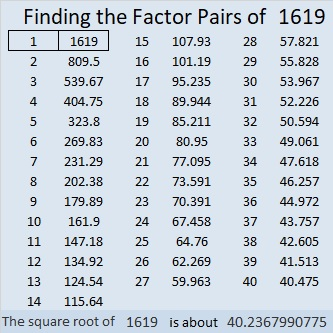# 1619 A Pink Egg Hidden in the Grass

### Today’s Puzzle:

Easter is less than two weeks away. This pink puzzle is the first of three level-5 Easter eggs hidden amongst some blades of grass for you to find and solve. The puzzle might be a little tricky, but use logic every step of the way, and you’ll be able to find the unique solution:### Factors of 1619:

• 1619 is a prime number.
• Prime factorization: 1619 is prime.
• 1619 has no exponents greater than 1 in its prime factorization, so √1619 cannot be simplified.
• The exponent in the prime factorization is 1. Adding one to that exponent we get (1 + 1) = 2. Therefore 1619 has exactly 2 factors.
• The factors of 1619 are outlined with their factor pair partners in the graphic below.

How do we know that 1619 is a prime number? If 1619 were not a prime number, then it would be divisible by at least one prime number less than or equal to √1619. Since 1619 cannot be divided evenly by 2, 3, 5, 7, 11, 13, 17, 19, 23, 29, 31, or 37, we know that 1619 is a prime number.### More about the Number 1619:

1619 is the sum of two consecutive numbers:
809 + 810 = 1619.

1619 is also the difference of two consecutive squares:
810² – 809² = 1619.

What do you think about that?